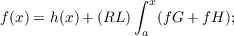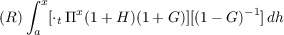#### Vol. 80, No. 1, 1979

 Recent Issues Vol. 322: 1  2 Vol. 321: 1  2 Vol. 320: 1  2 Vol. 319: 1  2 Vol. 318: 1  2 Vol. 317: 1  2 Vol. 316: 1  2 Vol. 315: 1  2Online Archive Volume: Issue:The Journal Subscriptions Editorial Board Officers Contacts Submission Guidelines Submission Form Policies for Authors ISSN: 1945-5844 (e-only) ISSN: 0030-8730 (print) Special Issues Author Index To Appear Other MSP Journals
Solution for an integral equation with continuous interval functions

### John Albert Chatfield

Vol. 80 (1979), No. 1, 47–57
##### Abstract

Suppose R is the set of real numbers and all integrals are of the subdivision-refinement type. Suppose each of G and H is a function from R ×R to R and each of f and h is a function from R to R such that f(a) = h(a), dh is of bounded variation on [a,x], and axH2 = axG2 = 0 for x > a. The following two statements are equivalent:

(1) If x > a, then f is bounded on [a,x], axH exists, axG exists, (RL) ax(fG + fH) exists, and(2) If a p < q x, then each of p Πq(1 + H) and p Πq(1 G)1 exists and neither is zero,exists, and

 f(x) = f(a)⋅a Πx(1 + H)(1 − G)−1 + (R)∫ ax[⋅ t Πx(1 + H)(1 + G)][(1 − G)−1]dh.

##### Mathematical Subject Classification 2000
Primary: 45N05
Secondary: 26A42, 44A40
##### Milestones